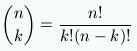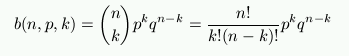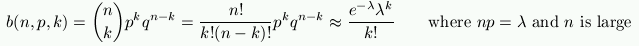### Math 210, Spring 2001: Day By Day Part 1

A day by day outline of some topics what we cover.

These are not intended to be balanced or comprehensive notes. They are to supply motivation and fill gaps. Thus, standard material easily available in traditional texts will be skipped, while brief (quick and dirty) notes about computer or Internet issues may be here.

In principle, the Wednesday classes will involve more computer issues. But real life is never that tidy.

Contents

Tues. Jan. 16
Outline of the course. Some elementary probability.
If you roll two dice, what is the likelihood of at least one face being a 2? What is the likelihood of the sum being 5?
Birthday Problem: There are 25 people in a room. What is the likelihood that at least two of them have the same birthday? What is the probability P that exactly two of them have the same birthday? The new idea in this problem is that computing probabilities involving  or  is usually much more complicated than those using  and . We compute the probability Q that none of the 25 people have the same birthday. Then P = 1 - Q.
One reference for this lecture is Chapters 1 and 3 of M. Grinstead, Charles M. and J. Laurie Snell, Introduction to Probability. See Math 210 Bibliography for pdf files with these chapters. If you find these useful, you may want to buy the printed book.

Wed. Jan. 17
Writing your own web "Home Page". How do you give others permission to read a web page you wrote? Where do you put these pages on your computer?
A Simple Web Page   A More Complicated Example.
A Few Unix commands
(to be added: copying files and moving them to other directories)

Thurs. Jan. 18
Carrying out the computation in the Birthday Problem. We need to compute (364/365)(363/365)...(341/365). This can be painful. We write a computer program using perl Birthday Computation program for 50 people
If you roll one die 4 times, what is the probability of getting at least one 6?
If you roll two dice 17 times, what is the probability of getting at least one pair of 6's?

Tues. Jan. 23
Permutations: Say you have the letters A, B, C, D, and E. How many different three letter "words" can you make using each letter exactly once? Thus here the order of the letters is essential; the word "BAD" is not the same as "DAB".
General Answer: If you have n "letters" there are n!/(n-k)! words with exactly k letters. For this example there are 5!/(5-3)! =60. words.

Indistinct Objects: The same question, only now the order of the k letters is irrelevant. Since there are 3! ways of rearranging three letters, now there are only 60/3! = 10 sets with three different letters.
General Answer: If you have n "letters" there are n!/[k!(n-k)!] sets with exactly k letters. This is is the binomial coefficient:If you expand (1+x)n = 1 + nx + ... , this is the coefficient of xk.

Binomial Probabilities: A biased coin is tossed five times. Say there is a probability p of heads and thus q=(1-p) of tails. Say you win if exactly three of the tosses give heads. Thus you win with any the sequences HHHTT, HHTHT, etc.
There is a probability of p3q2 of the winning sequences HHHTT, and similarly for any of the other winning sequences HHTHT, etc. Since from the previous paragraph there there are 5!/[3!(5-3)! = 10 different winning sequences, the probability of winning is 10p3q2.

In general with n trials, each having a success probability p, the probability of exactly k successes isThis situation is called a Bernoulli Trial. It is a sequence of n experiments, each with only two possible outcomes, usually named success with probability p and failure with probability q = 1-p. The probability of success on any experiment is assumed to be independent of the previous experiments.

See Grinstead and Snell Chapter 3 Grinstead and Snell, Chapter 3 (pdf)

Wed. Jan. 24
To transfer files between computers, one standard method is to use FTP. A simple version comes on almost all computers. A slicker windowing program is WS FTP. Penn students can download it for free: WS FTP

Thurs. Jan. 25
Day of the Week: Given a month, day, and year, how do you compute the day of the week? Pick a reference day, say Jan. 1, 2001 which was a Monday. Find how many days have elapsed between then and your target date. Thus, if 14 or 700 days have elapsed, then since these numbers are divisible by 7, the given date is also a Monday. If 702 days have elapsed it is a Wednesday.
Example: June 1, 2001 We need to observe that June 1 is the 152nd day in 2001 (since 31+28+31+30+31+1 = 152) so it is 152-1 = 151 days after Jan. 1. Because 151 = 21*7 + 4, we know the day is 4 days later, a Friday.
Example: June 1, 2017 We know that 16 full years have elapsed making 16*365 days, to which we need to add 4 days for the leap years 2004, 2008, 20012, and 2016. Finally, as above we add 152-1=151 to compute that N = 16*365 + 4 + 151 days have elapsed since Jan. 1, 2001. To determine the day of the week we need only the remainder when dividing N by 7. It is 3 so the day is three days after Monday: a Thursday.
To check these computations, on a Unix computer the command cal 2017 gives a calendar for the year 2017, while cal 6 2017 gives a calendar for June 2017.

Tues. Jan. 30
If you want to post something on the web, where on your computer do you put it? The answer depends on how the computer was set-up. On mail.sas, eniac.seas and the class computer johnny.sas files you have go in the directory named html, a subdirectory of your "home" directory. They can also go in sub-directories of this directory.
Although most computers do not allow you to have programs on the web, our class does allow this. However, for security, they can only be in the directory html/cgi-bin/ (or its sub-directories).
Any programs on the web must allow anyone to execute them. On a Unix computer this usually means
chmod 755 [program_name]

Programs not intended for the web can be located in any of your directories. You may wish to make a directory 210 for our class stuff:
`  mkdir \$HOME/210`
Although we will primarily use Perl in class, please feel free to use any other computer language you may prefer. Just don't assume that I will be able to help you with it.

A simple Perl example. This will also run on mail.sas or eniac.seas, except that you may need to change the location of perl. [To find the location just type which perl.]

A Web form that collects input for an addition Perl program. This is essentially identical to the previous line, except using the Web for input.
Another example using the Web for input

A similar script, only using JavaScript instead of Perl. This is entirely self-contained. View the Page Source to see the details.

See Voting on the Web for a sequence of Perl examples I wrote for this class.

Wed. Jan. 31
In a Perl program, how do you supply input? In the simple example perl_example0 the values of the numbers to be added were simply typed in the program. Here are two more graceful methods:
command line input: program command line
Copy these programs to your own directory and try them. Remember to change the permissions so these programs are executable:   chmod 755 [name_of_program] .
Some Perl examples:
• test for equality of numbers: if (\$x ==3 ) {\$y = 2 };
• test for equality of strings:
if (\$name eq "Brian") {print "\n\tHi \$name\n"; }
• integer division remainder: (19 % 4) gives the remainder when dividing 19 by 4.
• integer part of a number:  int(17.34) gives 17 .
Note int(-17.34) gives -17, which may not be what you want (int always rounds toward 0). Use floor to get rounding to the next lower integer. Thus floor(-17.34) gives -18. Since floor is not a standard part of perl, before using it you need to have one line with the following:
use POSIX "floor";
• Random numbers. \$x = rand(6) assigns \$x a random real number 0 <= \$x < 6 .
To simulate random heads/tails you can use \$toss = int(rand(2)), where 0 means Heads and 1 Tails.
To simulate a roll of a die, try \$roll = int(rand(6)) +1.

Conditional probability (more). A tree diagram is often useful when analyzing a situation involving conditional probability. See the discussion in Section 4.1 of Grinstead and Snell, Chapter 4 (pdf)

Thursday, Feb. 1
Computer simulation. Frequently it is helpful to use the computer to simulate some experiment. Here is a simple one for tossing a coin. We toss a coin 5000 times and record how many Heads there are. Coin Toss Simulation

Day of the week. Here is one version. It asks for the date. Day of Week (perl)
If one cleans up, this appears much shorter (but less clear): Day of Week

The computation of binomial probabilities (see Jan. 23)can be painful for n large. Poisson found a useful approximation for large n and small p, that is, if we let n get very large but keep np fixed. His approximation is:In class we used this to compute the answer in an application where n =12,000 and p = 1/8,000 --- in which case the approximation is excellent.

Tuesday, Feb. 6
Today we discussed the "Bus Problem" (see the homework) as an entry to the topic continuous probability distributions. This is treated in all probability books.

Wednesday, Feb. 7
Discussion of some of the homework problems.
Computer graphics using matrices -- and an example using Maple: The Letter F (see also F page 1 and F page 2).